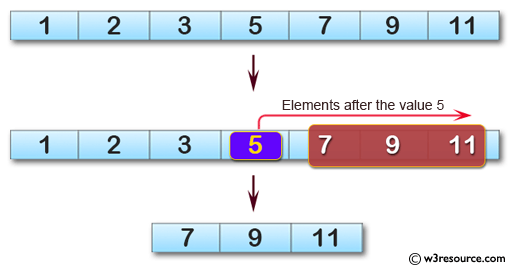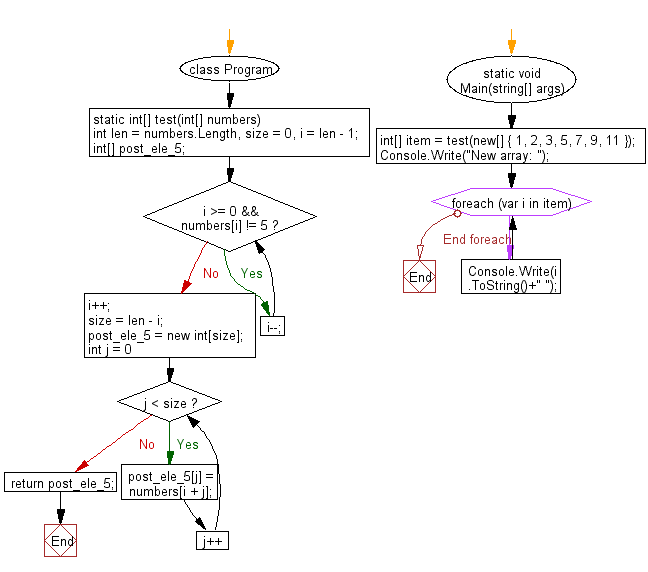﻿ C# - New array of the values after the value 5 from a array# C# Sharp Basic Algorithm Exercises: Create a new array taking the elements after the element value 5 from a given array of integers

## C# Sharp Basic Algorithm: Exercise-129 with Solution

Write a C# Sharp program to create a new array taking the elements after the element value 5 from a given array of integers.

Pictorial Presentation:Sample Solution:

C# Sharp Code:

``````using System;
namespace exercises
{
class Program
{
static void Main(string[] args)
{
int[] item = test(new[] { 1, 2, 3, 5, 7, 9, 11 });
Console.Write("New array: ");
foreach(var i in item)
{
Console.Write(i.ToString()+" ");
}
}
static int[] test(int[] numbers)
{
int len = numbers.Length, size = 0, i = len - 1;
int[] post_ele_5;

while (i >= 0 && numbers[i] != 5) i--;
i++;

size = len - i;
post_ele_5 = new int[size];

for (int j = 0; j < size; j++)
{
post_ele_5[j] = numbers[i + j];
}

return post_ele_5;
}
}
}
```
```

Sample Output:

```New array: 7 9 11
```

Flowchart:C# Sharp Code Editor:

Improve this sample solution and post your code through Disqus

What is the difficulty level of this exercise?

Test your Programming skills with w3resource's quiz.

﻿